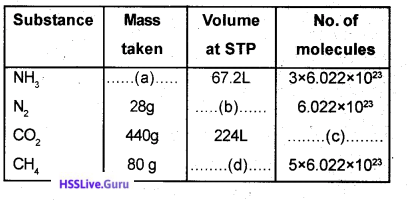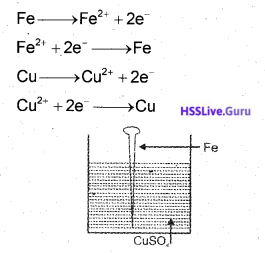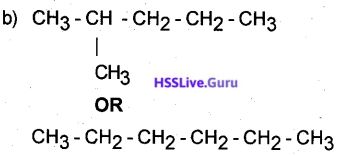# Kerala SSLC Chemistry Model Question Paper 3 English Medium

Students can Download Kerala SSLC Chemistry Model Question Paper 3 English Medium Pdf, Kerala SSLC Chemistry Model Question Papers helps you to revise the complete Kerala State Board New Syllabus and score more marks in your examinations.

## Kerala SSLC Chemistry Model Question Paper 3 English Medium

General Instructions:

1. The first 15 minutes is the cool off time. You may use the time to read and plan your answers.
2. Answer the questions only after reading the instructions and questions thoroughly.
3. Questions with marks series 1, 2, 3 and 4 are categorized as sections A, B, C and D respectively.
4. Five questions are given in each section. Answer any four from each section.
5. Answer each question by keeping the time.

Time: 1½ Hours
Total Score: 40 Marks

Section – A

(Answer any 4 questions from 1 to 5. Each question carries 1 score) (4 × 1 = 4)

Question 1.
Which one of the following electron configuration is not correct?
a) 1s22s23s2
b) 1s22s2
c) 1s22s22p6
d) 1s22s22p63s1
a) 1s22s23s2Question 2.
The volume of 5 mol ammonia (NH30 at STP will be …….
a) 5L
b) 22.4L
c) 17L
d) 112L
1121

Question 3.
The metal that cannot liberate hydrogen when react with dilute hydrochloric acid is …….
a) Copper
b) Magnesium
c) Zinc
d) Aluminium
CopperQuestion 4.
To which category the compound CH3-COO-CH3 belongs to?
a) Carboxylic acid
b)Ethene
c) alcohol
d) Ester
Ester

Question 5.
Which of the following substance is used to identify a sulphate salt?
a) Hydrochloric acid
b) Silver nitrate
c) Barium chloride
d) Liquor Ammonia
Barium chloride

Section-B

(Answer any 4 questions from 6 to 10. Each question carries 2 scores)(4 × 2 = 8)

Question 6.
From the statements given below select those applicable to ‘s’ block elements.
a) high electronegativity
b) high ipnisation energy
c) loses electrons in chemical reactions
d) forms acidic oxides
Loses electrons in chemical reactions.Question 7.
Complete the blanks in the table given below.a) 17 × 3 = 51 g
b) 22.4 L
c) 10 × 6.022 × 1023
d) 22.4 × 5 = 112L

Question 8.
An iron nail is immersed in copper sulphate solution.
a) What will be the observation after some time?
b) Which of the following reactions include in this change?a) Iron nail will be coated with copper
b) Fe → Fe2+ + 2e
Cu2+ + 2e → CuQuestion 9.
Match those given in the columns A, B and C suitably.

 A B C Blast furnance Manufacture of Aluminium Reduction using CO Hall-Heroult Concentration process of the ore Electrolysis Calcination Manufacture of iron Calamine

Blast Furnace – Production of Iron – Reduction using CO
Hall – Heroult process – Production of Aluminium – Electrolysis
Calcination-Concentration of ore-Calamine

Question 10.
Find out a pair of isomers from those given below. Also write what type of isomers they are?
a) CH3-CH2-CH2-OH
b) CH3-CH2-CH2-CH3
c) CH3-O-CH2-CH3
d) CH3-CH2-CH2-CH2-OH
CH3-CH2-CH2-OH and CH3-O-CH2-CH3 Funtional group isomers

Section – C

(Answer any 4 questions from 11 to 15. Each question carries 3 scores) (4 × 3 = 12)

Question 11.
The subshell electron configuration of two ions are given below. (Symbols are not real)
X2+ = 1s22s22p6
Y1- = 1 s22s22p6
a) Write the subshell configuration of the elements X, and Y.
b) Write the chemical formula of the compound formed by the elements X and Y.
a) X – 1s22s2 2p63s2
Y – 1s22s22p5
b) XY2Question 12.
The atomic mass of oxygen is 16. Match those in – the following table suitably.

 A B C 16 g Oxygen 1GMM 6.022 × 10 23 molecules 160 g Oxygen 1 GAM 6.022 × 1023 atoms 32 g Oxygen 5 mole 112 L volume at STP

16g Oxygen – 1 GAM – 6.022 × 1023 atoms
16g Oxygen – 5 mol – 112 L volume at STP
32g Oxygen – 1 GMM – 6.022 × 1023 molecules

Question 13.
Write the products obtained in the following circumstances.
a) Molten NaCl is electrolysed.
b) Aqueous solution of NaCl is electrolysed
a) Molten NaCI
At anode – Chlorine
At cathode – Sodium

b) Aqueous solution of NaCI
At anode-Chlorine
At cathode-Hydrogen.Question 14.
The different stages involved in the concentration of the ore Of aluminium is given as a flow chart. Complete the missing columns.
A – Sodium aluminate – NaAlO2
B – Aluminium hydroxide-AI(OH)3
C – Alumina -Al2O3

Question 15.
a) Complete the following equations by writing the formula/name of the products.b) Write balanced equation for the combustion of the fuel propane'(C3H8).Section – D

(Answer any 4 questions from 16 to 20. Each question carries 4 scores)(4 × 4 = 16)

Question 16.
Analyse the structural formula of a Compound given below.a) Write the IUPAC name of this compound.
b) Write the structural formula of a possible isomer of this compound.
c) Which type of isomers these compounds are?
a) 2,3-dimethyl butanec) Chain isomersQuestion 17.
The alloys Nichrome and Alnico contains same components.
a) Which are the component elements in these alloys?
b) Write any one use of each alloy.
c) Why does these alloys differ in properties eventhough they contain same components?
a. Al, Ni, Cr, Fe
b. Alnico – used to omake magnets Nichrome – used to makeJieating coil of heating appliances.
c. The ratio of components is different

Question 18.
The equation of a reversible reaction is given below.
N2(g) + 3H2(g) ⇌ 2NH3(g) + Heat
What is the influence of the following factors?
a) Increases pressure
b) Increases the concentration of N2
c) Ammonia (NH3) is continuously removed from the sytem.
d) A catalyst is used.
a) Forward reaction increases
b) Forward reaction increases
c) Forward reaction increases
d) Both forward and backward reaction increases and equillibrium state is attained at an early stage.

Question 19.
Write the observation and the reason for it in the following experiments.
a) Barium chloride (BaCl2) is added to the solution of a sulphate salt.
b) Cone. H2SO4 is added to cane sugar crystals.
c) Cone. H2SO4 is added to water taken in a beaker.
d) Cone. H2SO4 is poured in a dry paper.
a. A white precipitate is formed. Sulphate salts reacts with Barium chloride to form white Barium sulphate.
b. Sugar changes to sugar charcol. Con. H2SO4 absorbs hydrogen and oxygen present in sugar in the ratio as that of water. (2:1)
c. Heat is liberated. Dissolution of H2SO4 in water is exothermic!
d. Paper get charred. Dehydration property of H2SO4Question 20.
a) How ethanol is manufactured on a large scale?
b) Write any two uses of ethanol
c) What is power alcohol?
d) What is methylated spirit?